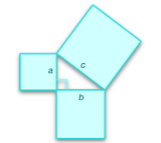# Ryangineer

## Machine LearningAlgorithms

"The question of whether a computer can think is no more interesting than the question of whether a submarine can swim."
Edsger W. Dijkstra

### Noteworthy Machine Learning Algorithms

Machine Learning   ⇒   software able to detect patterns, make decisions, predict outcomes, learn from mistakes & optimize own performance without being explicitly programmed to do so

#### Supervised Learning

↳ "learning a function that maps to an output based on the example of input-output pairs"
• ##### Linear Regression | Predict Real Values
Estimate or predict real values based on continuous variables -> establish relationship between independent variables (matrix of features) & dependent variable (output) by fitting a best line
• ##### Homoscedasticity
"Homoskedastic . . . refers to a condition in which the variance of the residual, or error term, [that is, the “noise” or random disturbance in the relationship between the independent variables and the dependent variable], in a regression model is constant. That is, the error term does not vary much as the value of the predictor variable changes." Investopedia
• ##### Multicollinearity
"[R]efers to predictors that are correlated [, that is, highly linearly related,] with other predictors. Multicollinearity occurs when your model includes multiple factors that are correlated not just to your response variable, but also to each other. In other words, it results when you have factors that are a bit redundant." Minitab
• ##### No Free Lunch Theorems (NFL)
"[S]tate that any one algorithm that searches for an optimal cost or fitness solution is not universally superior to any other algorithm. . . . 'If an algorithm performs better than random search on some class of problems then in must perform worse than random search on the remaining problems.'” Medium
• ##### Parsimonious Model
"Parsimonious models are simple models [with the least assumptions & variables but] with great explanatory predictive power. They explain data with a minimum number of parameters, or predictor variables. The idea behind parsimonious models stems from Occam's razor, or 'the law of briefness' (sometimes called lex parsimoniae in Latin)." Statistics How To
• ##### Simple Linear Regression
Combining one variable in an equation to predict a single outcome
• ##### Multiple Linear Regression
Combining many variables in an equation to predict a single outcome
• ##### Support Vector Regression | Classification
Use as a regression method, maintaining all the main features that characterize the algorithm (maximal margin). The Support Vector Regression (SVR) uses the same principles as the SVM for classification, with only a few minor differences.
• ##### Logistic Regression | Classification
Used to estimate discrete values, binary values (0/1, yes/no, true/false) based on given set of independent variables; predicts probability between 0 & 1 as output values.
Logistic regression like its name is logarithmic. Its graph is curvilinear. If the dependent variable is binary, the graph is sigmoid. If not, the graph can be more pronounced, parabolic, etc.
• ##### Decision Tree Regression
Supervised learning algorithm used for classification problems; works for categorical & continuous variables
• ##### Support Vector Machines | Discriminative Classifier
Discriminative classifier formally defined by a separating hyperplane
• ##### Kernel SVM | Nonlinear
• Mapping to a higher-dimensional space, applying the support vector algorithm & then projecting back to lower dimensional space resulting in a nonlinear separator
• ##### Naive Bayes Classification
Probabilistic classifier based on Bayes Theorem with an assumption of independence between predictors (aka, features or independent variables)
• ##### Bayes Theorem ⇒ The probability of an event given prior knowledge of related events that occurred earlier
$P\left(y\mid {x}_{1},\dots ,{x}_{n}\right)=\frac{P\left(y\right)P\left({x}_{1},\dots ,{x}_{n}\mid y\right)}{P\left({x}_{1},\dots ,{x}_{n}\right)}$
• ##### K-Nearest Neighbors
Used for classification & regression; a simple algorithm that stores all available cases & classifies new cases by a "majority vote" of its K-nearest neighbors
• ##### Euclidean Distance
$\mathrm{Between}{P}_{1}&{P}_{2}=\sqrt{\left({x}_{2}-{x}_{1}\right){2}^{}+\left({y}_{2}-{y}_{1}\right){2}^{}}$

#### Unsupervised Learning

↳ "looks for previously undetected patterns in a data set with no pre-existing labels and with a minimum of human supervision"

#### Reinforcement Learning

↳ "how software agents ought to take actions in an environment in order to maximize the notion of cumulative reward"

### Lovely Deep Learning

#### Artificial Neural Networks

↳ A computing system that consist of a number of simple but highly interconnected elements or nodes, called ‘neurons’, which are organized in layers which process information using dynamic state responses to external inputs, an extremely useful algorithm for finding patterns too complex to be manually extracted

#### Convolutional Neural Networks

↳ A class of deep neural networks, most commonly applied to analyzing visual imagery. CNNs are regularized versions of multilayer perceptrons. Multilayer perceptrons usually mean fully connected networks, that is, each neuron in one layer is connected to all neurons in the next layer.

#### Natural Language Processing

↳ Starts with raw text in whatever format available, processes it, extracts relevant features and builds models to accomplish various NLP tasks
• ##### Document-Term Matrix
Compute dot product (sum of the products of corresponding elements) to find similarities
• ##### Cosine Similarity
Divide the product of two vectors by their magnitudes or Euclidean norms
• ##### TF-IDF Transform
Term frequency-inverse document frequency
• ##### Stemming
Takes the root of a word removing conjugation to simplify & understand gist meaning (reducing final dimension )
• ##### Lemmatization
Refers to doing things properly with the use of a vocabulary and morphological analysis of words, normally aiming to remove inflectional endings only and to return the base or dictionary form of a word, which is known as the lemma.